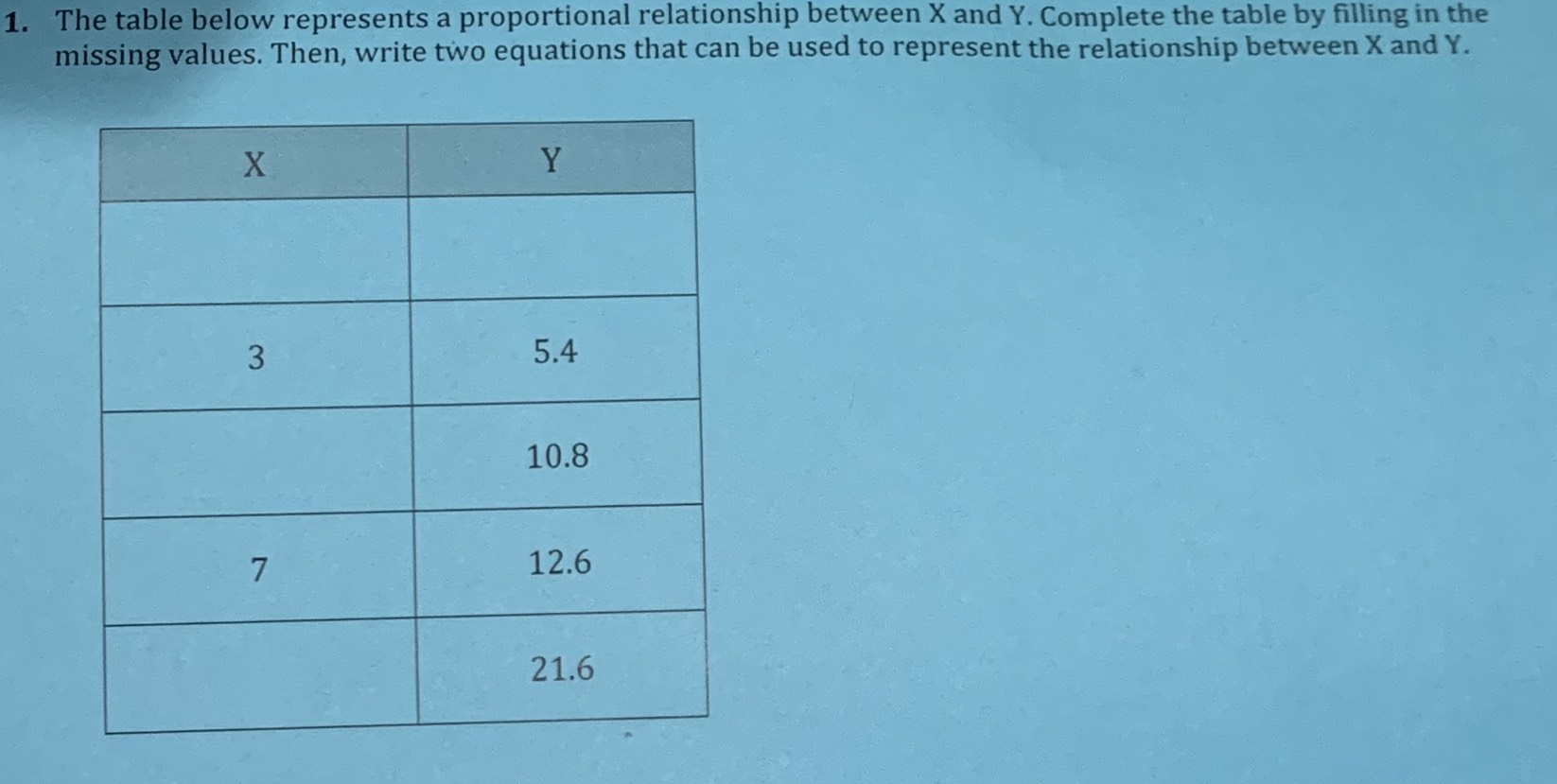### ¿Todavía tienes preguntas de matemáticas?

Pregunte a nuestros tutores expertos
Algebra
PreguntaThe table below represents a proportional relationship between $$X$$ and $$Y$$ . Complete the table by filling in the missing values. Then, write two equations that can be used to represent the relationship between X and Y.